• ## 二项式定理

千次阅读 2018-08-14 11:07:10
二项式定理，又称牛顿二项式定理，此定理给出两个数之和的整数次幂诸如展开为类似项之和的恒等式。二项式定理可以推广到任意实数次幂，即广义二项式定理  可以将x+y的任意次幂展开成和的形式 其中每个   为...


二项式定理

二项式定理，又称牛顿二项式定理，此定理给出两个数之和的整数次幂诸如展开为类似项之和的恒等式。二项式定理可以推广到任意实数次幂，即广义二项式定理

可以将x+y的任意次幂展开成和的形式其中每个为一个称作二项式系数的特定正整数，其等于。这个公式也称二项式公式或二项恒等式。使用求和符号，可以把它写作展开全文• 二、组合恒等式 ( 变下项求和 ) 变系数求和 1 证明 ( 二项式定理 + 求导 ) 、 三、组合恒等式 ( 变下项求和 ) 变系数求和 2 、 四、组合恒等式 ( 变下项求和 ) 变系数求和 2 证明 ( 使用已知恒等式证明 )
文章目录一、组合恒等式 ( 变下项求和 ) 变系数求和 1二、组合恒等式 ( 变下项求和 ) 变系数求和 1 证明 ( 二项式定理 + 求导 )三、组合恒等式 ( 变下项求和 ) 变系数求和 2四、组合恒等式 ( 变下项求和 ) 变系数求和 2 证明 ( 使用已知恒等式证明 )

一、组合恒等式 ( 变下项求和 ) 变系数求和 1

组合恒等式 ( 变下项求和 ) 变系数求和 :
$\sum_{k=0}^{n} k \dbinom{n}{k} = n 2^{n-1}$

$k$ 随着求和的项不断变化 , 变化范围 $0$ ~ $n$ ;

1. 证明方法 :

二项式定理 : 使用 二项式定理 + 求导 可以证明该组合恒等式 ;
组合恒等式代入 :  使用 已知组合恒等式代入 , 消去变系数  ; 即使用之前的 $3$ 个递推式 , 简单和 , 交错和 , $5$ 个组合恒等式 代入 ;

二、组合恒等式 ( 变下项求和 ) 变系数求和 1 证明 ( 二项式定理 + 求导 )

使用二项式定理 + 求导方法证明下面的恒等式 :
$\sum_{k=0}^{n} k \dbinom{n}{k} = n 2^{n-1}$

二项式定理 : $(x + y)^n = \sum\limits_{k=0}^n \dbinom{n}{k}x^k y^{n-k}$

1. $y = 1$ 时有该情况 :  $(x +1)^n = \sum\limits_{k=0}^n \dbinom{n}{k}x^k$ , 上述公式中 , 将常数项 $k= 0$ 的情况单独计算出来 , $\dbinom{n}{0}x^0 = 1$ , 计算过程如下 :
$(x +1)^n = \sum\limits_{k=0}^n \dbinom{n}{k}x^k = \dbinom{n}{0}x^0 + \sum\limits_{k=1}^n \dbinom{n}{k}x^k = 1+ \sum\limits_{k=1}^n \dbinom{n}{k}x^k$

2. 引入求导 : 要在加和式 $\sum\limits_{k=1}^n \dbinom{n}{k}x^k$ 中出现 $k$ 变化数 , 需要对 $x$ 进行求导 ;

这里直接对 $(x +1)^n = 1+ \sum\limits_{k=1}^n \dbinom{n}{k}x^k$ 等式两边进行求导 ;
( 1 ) 左边组合式 ( 根据下面的幂函数导数公式 计算 ) : $(x +1)^n$ 导数为 $n(x+1)^{n-1}$
( 2 ) 右边组合式 ( 根据下面的 导数运算规则 和 幂函数导数公式 计算 ) : $1+ \sum\limits_{k=1}^n \dbinom{n}{k}x^k$ 导数为 ,  $1$ 的导数 为 $0$ , 加上 $\sum\limits_{k=1}^n \dbinom{n}{k}x^k$ 的导数 $\sum\limits_{k=1}^n k \dbinom{n}{k}x^{k-1}$ , 最终结果是 $\sum\limits_{k=1}^n k \dbinom{n}{k}x^{k-1}$
( 3 ) 左右两边的导数是相等的 :
$n(x+1)^{n-1} = \sum\limits_{k=1}^n k \dbinom{n}{k}x^{k-1}$

幂函数求导 : ( 很重要 )

原函数 : $y = x^n$
对应导数 : $y' = nx^{n-1}$\

/
常数的导数是 $0$ ;
/
导数四则运算 : $(u \pm v)' = u' \pm v'$
/
参考 : 导数 - 百度百科

3. 求导后的结果如下 :
$n(x+1)^{n-1} = \sum\limits_{k=1}^n k \dbinom{n}{k}x^{k-1}$
假设求导结果中的 $x = 1$ ,  有如下结果 :
$n2^{n-1} = \sum\limits_{k=1}^n k \dbinom{n}{k}$
当 $k = 0$ 时 , 有 $k \dbinom{n}{k} = 0 \times \dbinom{n}{0} = 0$ ,
因此加上 $k=0$ 的情况 , 即 $k$ 从 $0$ 开始累加 , 也不影响上述结果 :
$n2^{n-1} = \sum\limits_{k=0}^n k \dbinom{n}{k}$

三、组合恒等式 ( 变下项求和 ) 变系数求和 2

组合恒等式 ( 变下项求和 ) 变系数求和 :
$\sum_{k=0}^{n} k^2 \dbinom{n}{k} = n ( n+1 ) 2^{n-2}$

$k$ 随着求和的项不断变化 , 变化范围 $0$ ~ $n$ ;

证明方法 :

二项式定理 : 使用 二项式定理 + 求导 可以证明该组合恒等式 ;
组合恒等式代入 :  使用 已知组合恒等式代入 , 消去变系数  ; 即使用之前的 $3$ 个递推式 , 简单和 , 交错和 , $5$ 个组合恒等式 代入 ;

四、组合恒等式 ( 变下项求和 ) 变系数求和 2 证明 ( 使用已知恒等式证明 )

使用 已知恒等式 证明下面的恒等式 :
$\sum\limits_{k=0}^{n} k^2 \dbinom{n}{k} = n ( n+1 ) 2^{n-2}$

1. 已知恒等式列举 :

① 递推式 $1$ : $\dbinom{n}{k} = \dbinom{n}{n-k}$
② 递推式 $2$ : $\dbinom{n}{k} = \dfrac{n}{k} \dbinom{n - 1}{k - 1}$
③ 递推式 $3$ 帕斯卡 / 杨辉三角公式 : $\dbinom{n}{k} = \dbinom{n - 1}{k} + \dbinom{n - 1}{k - 1}$
④ 变下项求和 1 简单和 : $\sum_{k=0}^{n}\dbinom{n}{k} = 2^n$
⑤ 变下项求和 2 交错和 : $\sum_{k=0}^{n} (-1)^k \dbinom{n}{k} = 0$

2. 变下限 : 从 $\sum\limits_{k=0}^{n} k^2 \dbinom{n}{k}$ 开始推导 , $k=0$ 时 , $k^2 \dbinom{n}{k} = 0$ , 可以忽略 , 这里可以从 $1$ 开始累加 ;

$\sum\limits_{k=0}^{n} k^2 \dbinom{n}{k} = \sum\limits_{k=1}^{n} k^2 \dbinom{n}{k}$

使用 $\dbinom{n}{k} = \dfrac{n}{k} \dbinom{n - 1}{k - 1}$ 恒等式替换其中的 $\dbinom{n}{k}$ :

$= \sum\limits_{k=1}^{n} k^2 \dfrac{n}{k} \dbinom{n - 1}{k - 1}$

3. 消去变系数 : 消去一个 $k$ 后 , 变成如下公式 :
$=\sum\limits_{k=1}^{n} k n \dbinom{n - 1}{k - 1}$

4. 常量外提 : 其中的 $n$ 相对于求和来说 , 是一个常量 , 可以提到求和符号之外 :

$=n\sum\limits_{k=1}^{n} k \dbinom{n - 1}{k - 1}$

5. 变形及拆解 : 在组合数中有 $\dbinom{n - 1}{k - 1}$ , 为了与 $k-1$ 进行匹配 , 这里将 $k$ 进行变形 , $k = (k - 1) + 1$ , 可以凑出一个 $k-1$ 来 ;

$=n\sum\limits_{k=1}^{n} [( k - 1 ) +1] \dbinom{n - 1}{k - 1}$

利用求和公式 , 将上述式子拆解成两个和式 ,

$=n\sum\limits_{k=1}^{n} ( k - 1 ) \dbinom{n - 1}{k - 1} + n\sum\limits_{k=1}^{n} \dbinom{n - 1}{k - 1}$

6. 第一个组合式转换 : $n\sum\limits_{k=1}^{n} ( k - 1 ) \dbinom{n - 1}{k - 1}$ 求和 ,
$k=1$ 时 , 组合数的下项 , 加和式中的系数 $k-1=0$ , 将 $k$ 作下限的变换 , $k$ 取值是 $1$ ~ $n$ , 则 $k-1$ 取值是 $0$ ~ $(n-1)$ ,
相当于使用 $k' = k-1$ 替代之前的 $k$ , $k'$ 取值范围 $0$ ~ $(n-1)$ ,
因此最终可以变为 $n\sum\limits_{k'=0}^{n-1} ( k' ) \dbinom{n - 1}{k'}$
使用 $\sum\limits_{k=0}^{n} k \dbinom{n}{k} = n 2^{n-1}$ 组合恒等式 ,
上述 $\sum\limits_{k'=0}^{n-1} ( k' ) \dbinom{n - 1}{k'}$ 的结果是 $(n-1)2^{n-2}$ ,
前面乘以 $n$  , 最终的 $n\sum\limits_{k'=0}^{n-1} ( k' ) \dbinom{n - 1}{k'} = n(n-1)2^{n-2}$

7. 第二个组合式转换 : $n\sum\limits_{k=1}^{n} \dbinom{n - 1}{k - 1}$ 该组合式中 $k$ 取值是 $1$ ~ $n$ , 将 $k$ 变为从 $0$ 开始 , 即使用 $k' = k-1$ 替代 $k$ ,
则有 $n\sum\limits_{k'=0}^{n-1} \dbinom{n - 1}{k'}$ , 该求和可以转变成基本求和 ,
使用 简单和 组合恒等式 $\sum\limits_{k=0}^{n}\dbinom{n}{k} = 2^n$ ,
$\sum\limits_{k'=0}^{n-1} \dbinom{n - 1}{k'}$ 就是 $2^{n-1}$ , 前面乘以 $n$ , $n\sum\limits_{k=1}^{n} \dbinom{n - 1}{k - 1}$ 就是 $n2^{n-1}$

$=n\sum\limits_{k=1}^{n} ( k - 1 ) \dbinom{n - 1}{k - 1} + n\sum\limits_{k=1}^{n} \dbinom{n - 1}{k - 1}$
$=n(n-1)2^{n-2} + n2^{n-1}$
$=n(n-1)2^{n-2} + n 2 \times2^{n-2}$
$=n(n+1)2^{n-2}$

总结 :
先利用 递推式 , 消掉变系数 $k$ ,
将求和的每个式子凑成基本求和公式 , 或 已知求和公式 ,
然后进行求和变限 , 即使用 $k' = k-1$ 替换 $k$ ,
通过上述技巧 , 完成证明 ;


展开全文• 数列算术数列几何数列二项式定理数列、级数数列f(x)得到一个数列：2，4，6，8，...... ；数列是以自然数(1,2,3,4,.....)为定义域的函数对应值构成的序列--有限序列和无限序列。序列可以用函数表示，但通常我们用另一...
数列算术数列几何数列二项式定理数列、级数数列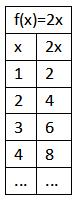f(x)得到一个数列：2，4，6，8，...... ；数列是以自然数(1,2,3,4,.....)为定义域的函数对应值构成的序列--有限序列和无限序列。序列可以用函数表示，但通常我们用另一种表示方式，如：f(x)=2x 可以写成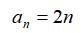序列通项公式有时我们知道数列中前几项，需要找到它的通项公式，即数列的数学模型。一般通项公式涉及到乘法、幂以及项的符号。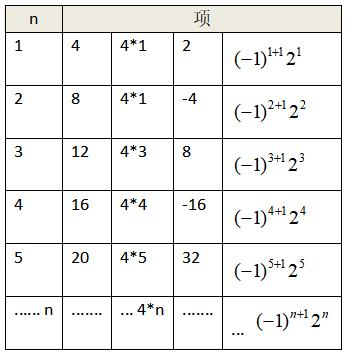阶乘序列是连续整数的乘积。如：5*4*3*2*1，即5！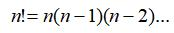n为正整数，且0!=1累加序列是项的累加。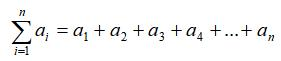算术数列算术数列是等差数列，即连续项之间的差为常数。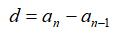d为常数等差数列的通项公式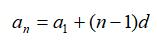a1、d 已知等差数列的求和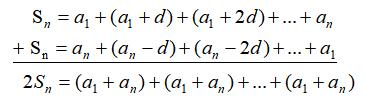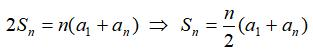等差数列求和公式几何数列几何数列是等比数列，即连续项之间的比例为常数。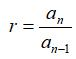r为常数等比数列的通项公式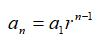a1、r 已知等比数列的求和公式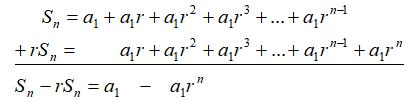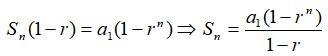|r|<1，无穷等比数列的和 a1/(1-r)|r|>1，无穷等比数列的和不存在，因为数列(级数)发散二项式定理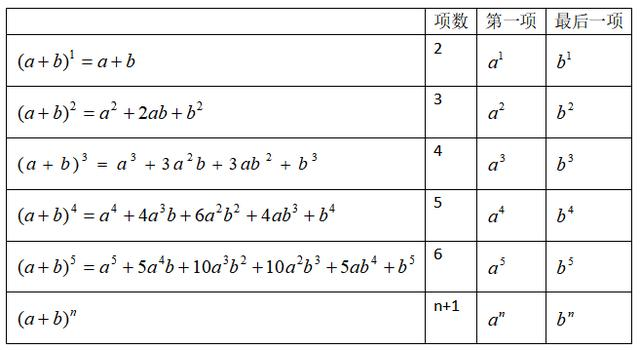通项式中，项数为n+1，各项的次数之和等于n，各项系数规律遵守帕斯卡三角形。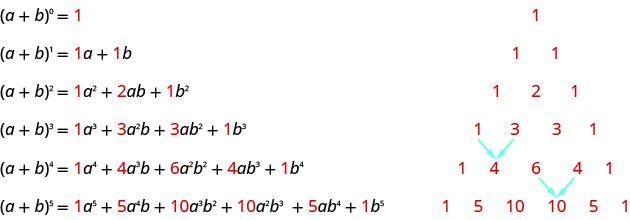二项式展开时，系数有两种方法得到帕斯卡三角形规则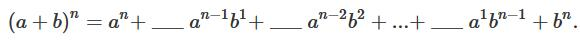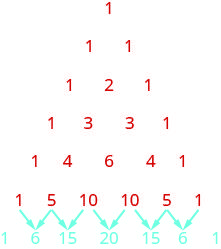第一行：次数n为0，后面以1为增量递增阶乘形式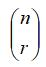从n个数里取出r个有多少种方法该方法应用在二项式展开得到系数，同样也可以用在概率上。其中，r、n都是整数且0≤r≤n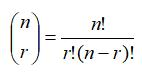二项式定理：a、b为实数，n为正整数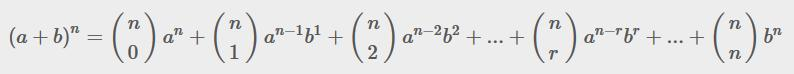展开全文• 二项式定理可以将x+y的任意次幂展开成和的形式 其中每个  为一个称作二项式系数的特定正整数，其等于 。 这个公式也称二项式公式或二项恒等式。使用求和符号，可以把它写作 很明显，当x==y==1时，会有下面这...


定理定义

二项式定理可以将x+y的任意次幂展开成和的形式其中每个为一个称作二项式系数的特定正整数，其等于。

这个公式也称二项式公式或二项恒等式。使用求和符号，可以把它写作很明显，当x==y==1时，会有下面这个公式

C(n,0)+C(n,1)+……C(n,n)=(1+1)^n=2^n

在容斥定理中：那么总共有多少项呢？其实就是组合数。就是2^n-1（因为里面没有C(n,0)这一项）
展开全文• 这种东西显然需要直接上斐波那契通公式。 设x=1+52,y=1−52,z=15x=\frac{1+\sqrt 5}{2},y=\frac{1-\sqrt 5}{2},z=\frac{1}{\sqrt 5}x=21+5​​,y=21−5​​,z=5​1​，则我们要求的就是这个东西： Ans=∑i=1nzk⋅...
• 题意：给定n个数a1,a2····an,依次求出相邻两个...例如n=3,m=2时，第一次得到a1+a2,a2+a3，在求和得到a1+2*a2+a3，它除以2的余数和a2无关。1=<n<=10^5, 2=<m<=10^9 Sample Input 3 2 Sample Ou...
• 给定n个数a1,a2····an,依次求出相邻两个数值和，将得到一个新数列，重复上述操作，最后结果将变为一个数，问这个数除以m的余数与那些数无关？例如n=3,m=2时，第一次得到a1+...　看一下 n 的范围是 10^5 , 在二项...
• 我对二项式定理(Binomial Theorem)的热爱无以言表，它看上去有很多数学符号，但本质上是用组合的方法来解决一个长得可怕的代数问题。尤其在你邂逅美妙的杨辉三角时，就会更感受到的数学不可思议之处。但当第一次遇到...
• 设www为kkk次单位根，由单位根求和引理和二项式定理，可以将我们需要求的东西转化为： Ans=∑i=0k−1(wi+1)nAns=\sum_{i=0}^{k-1}(w^i+1)^nAns=i=0∑k−1​(wi+1)n 代码： #include<iostream> #define ll ...
• （可恨的剪纸游戏） hdu多校第五场1009 这个规律是真的很讨厌，我比赛的时候撕了那么多的纸，结果却把自己绕了进去，还是哭唧唧 ...用到的知识就是二项式定理、快速幂和逆元 */ #include<bits/stdc
• 这道题没写过类似的感觉确实不好想，考点在与二项式求和优化等式。特别是优化等式这里，是真的坑。 废话不多说，上推到过程: 因为: 可以有: 所以有二项式展开: 很明显竖起来是等比，所以这样就可以把复杂度变成...
• 题意： 给定n个数a1，a2，...an,依次求出相邻两数之和，将得到一...例如n=3，m=2时，第一次求和得到a1+a2，a2+a3，再求和得到a1+2a2+a3，它除以2的余数和a2无关。 思路： 如果有n个数，最后结果就是杨辉三角的...
• 北大的这一道求和等式习题吸引我的注意。乍一看似乎很对称，甚至觉得理所应当。 那么实际上证明应该如何处理呢： 点击查看原文 如果觉得上面解答构造不易理解。我们尝试一步一步去解析： 证明： ∑k=0n(−1)kCnk1k...
• 直接用等比数列求和就可以证明了 而且在模$$998244353$$意义下的$$\omega_k^1=g^{P-1\over k}$$ 据说这玩意儿在$$NTT$$的证明里有？然而我那时候光顾着背板子了 所以这个单位根反演简称小单的玩意儿能干嘛...
• 复习一下数学, 找一下回忆. 先是从二项式平方开始: 其实展开是这样的: 再看立方: ...使用求和简写可得: ...数学常数 e (The Constant e – NDE/NDT Resource ...使用二项式定理能得出 第 k 项之总和为 因...
• 我们得到了自然数求和的通式： 我们既然找到了自然数的求和公式，就不妨再去推广一下，试着寻找一下推广的求和公式： 利用二项式定理计算 的封闭式为了算这个式子，我们不妨先研究一下这个求和式： 利用二项式定理，...
• 每次从1-n中抽取1个数，抽取后放回，共抽取b...可以得到求和公式，通过二项式定理化简后可得到简洁的表达式 #include #include #include #include #include #define INF 1 #include #define mem(a,b) memset(a,b,siz
• 二项式定理 这题要求的就是 $$\sum_{i=1}^n Fib(i)^k$$ ，其中 Fib 就是斐波那契数列 如果说没有 k 的话怎么做？仍然不会.jpg 于是我们直接想带 k 的答案吧... 我们考虑 把斐波那契数列的通项公式带进去！ 然后鬼都...
• 的Q的值，发现这样很难求，但带入f=x可以得到化简的一个利用二项式定理的启发，转化为Q=nx，考虑带入下降幂能发现原式被化简成n的i次下降幂*p的i次，之后考虑把f拆成下降幂形式，再在i位置乘上一个i！，就能发现下降...